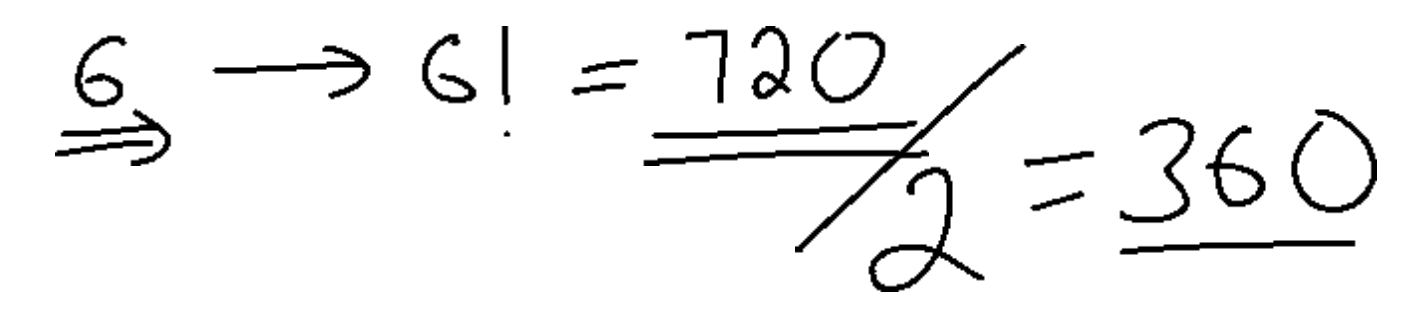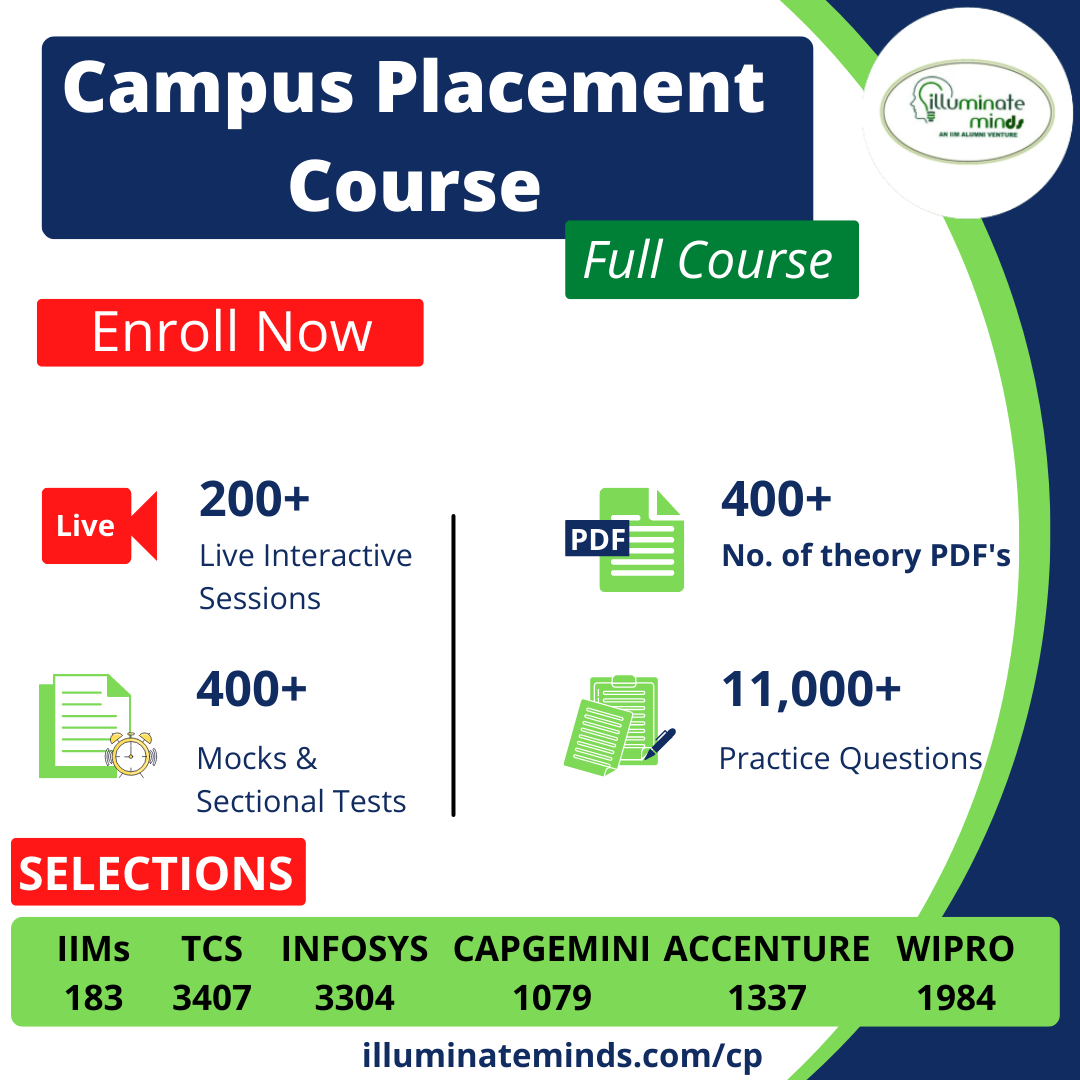Jobs By Batch
Jobs By Location
Jobs By Degree
Jobs By Branch
IT Jobs
Internships
Govt. Jobs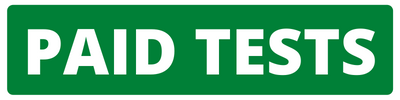• ##### Original Questions of IT Companies| All Topics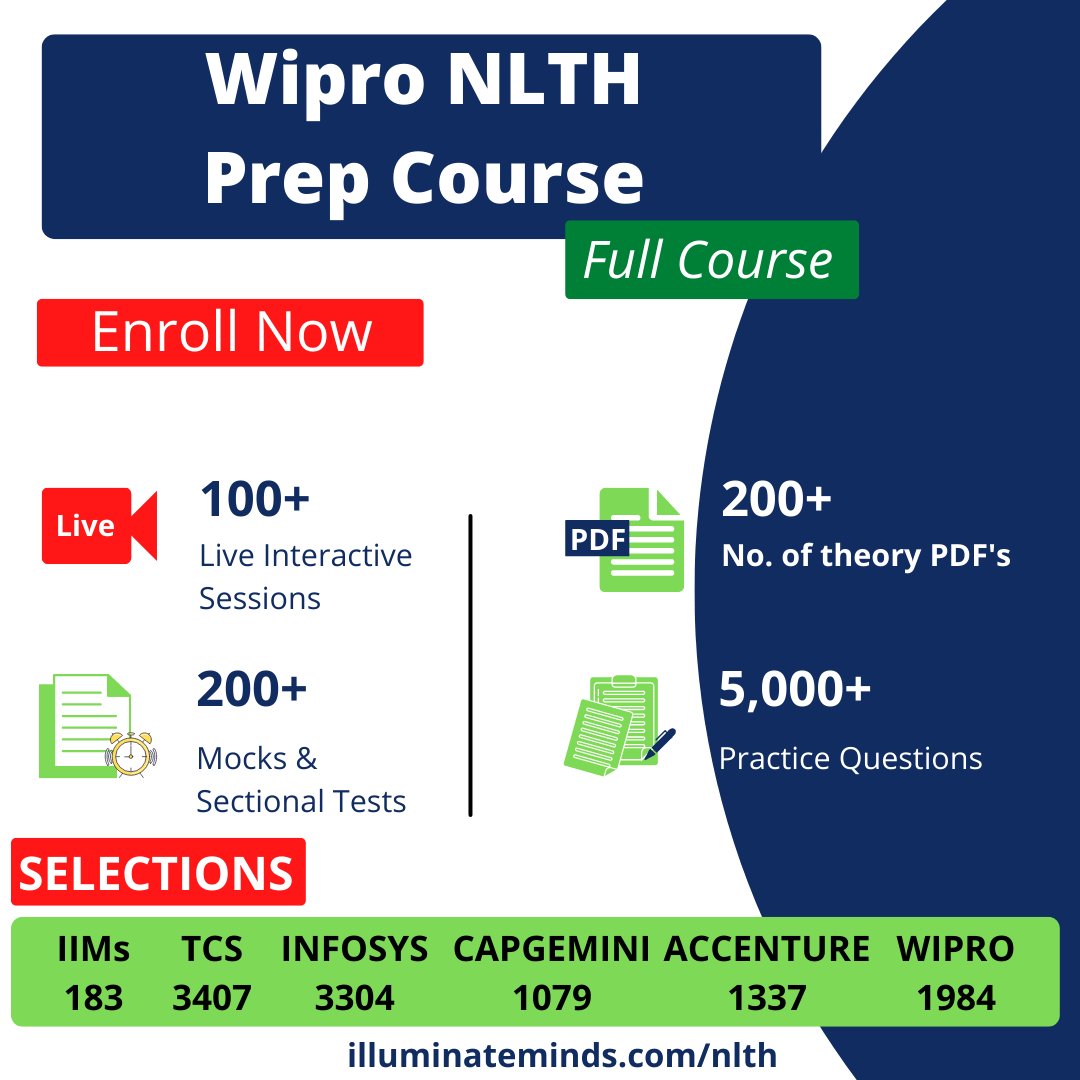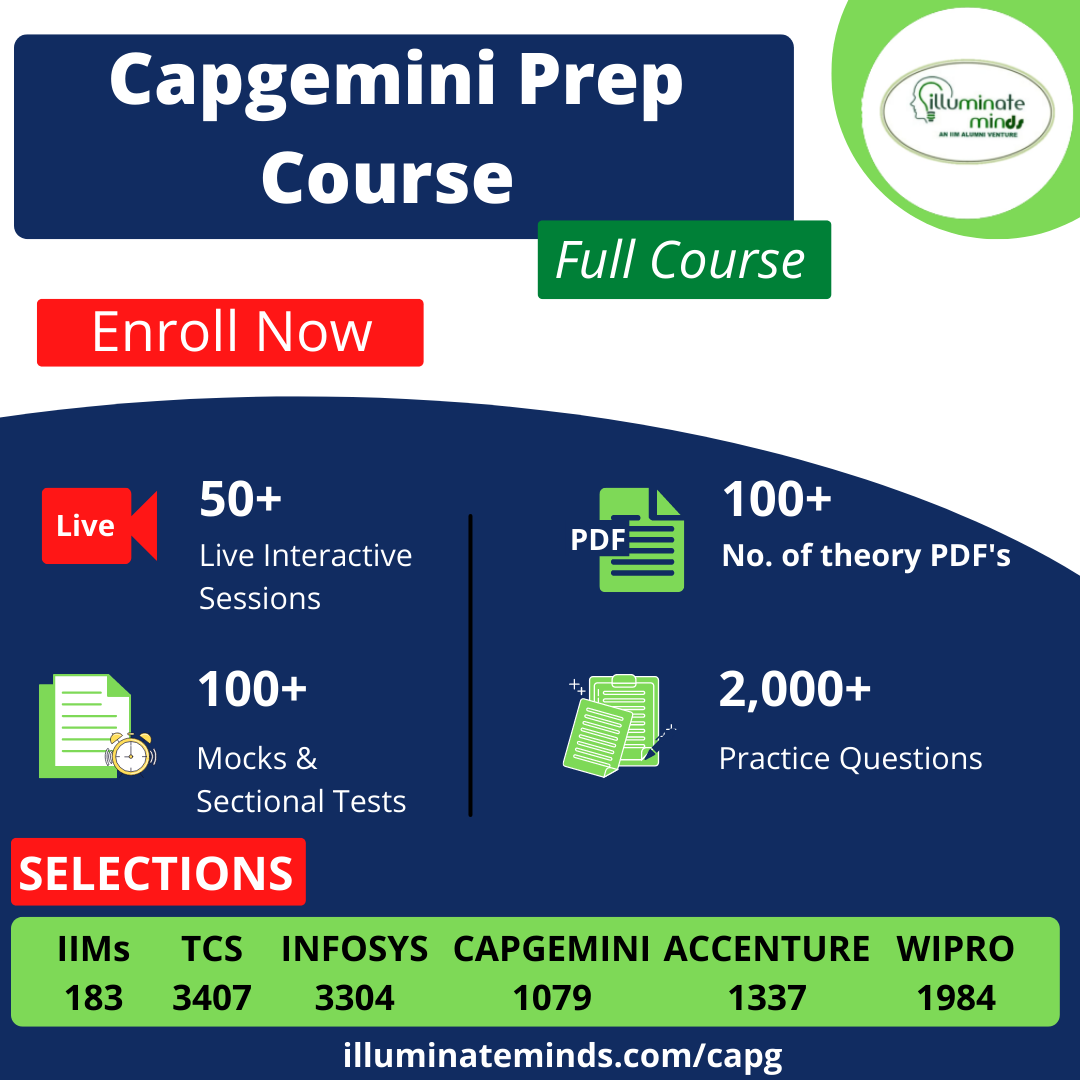Q #16
:

#### Quants Question

Two persons start, one from point A towards B and the other from B towards A. Distance between A and B is d meters. The person starting from B gives a whistle as soon as he starts and the person starting from A hears the whistle after 10 seconds of his (A) start. Speed of A and B are 2m/s and 1.5m/s respectively. Speed of sound is 330m/s.

What is the distance in metres?
+

Since A listens the whistle after 10 seconds, so both the sound of whistle

and person A both have travelled for 10 seconds, therefore the distance

travelled by A = 20 mts and that by sound = 330 × 10 = 3300 m. So the

distance d = 3320 m.

Q #17
:

#### Quants Question

Shiva and hari are asked to multiply a three-digit number M with a two-digit number N. Hari multiplied it correctly but Shiva reversed the digits of M as well as N and multiplied the number thus formed. Interestingly both of them got the same answer.
If the digits of M are distinct, What is the maximum possible value of N?
+

If digits of M are distinct then palindromes combinations are ruled out. So the maximum value of N is 81 (in the combination of 81 and 198).

Q #18
:

#### Quants Question

Ashish is given Rs 158 in one rupee denomination. He has been asked to allocate them into a number of bags such that any amount required between Re 1 and Rs 158 can be given by handing out a certain number of bags without opening them. What is the minimum number of bags required?
+

The coins should be put as follows: 1, 2, 4, 8, 16, 32, 64, 1, 2, 4, 8, 16, and hence he can meet all denominations. Hence 12 bags.

Q #19
:

#### Quants Question

Gold and No are in a horse race with 6contestants. How many different arrangements of finishes are there if No always finishes before Gold and if all of the horses finish the race?
+